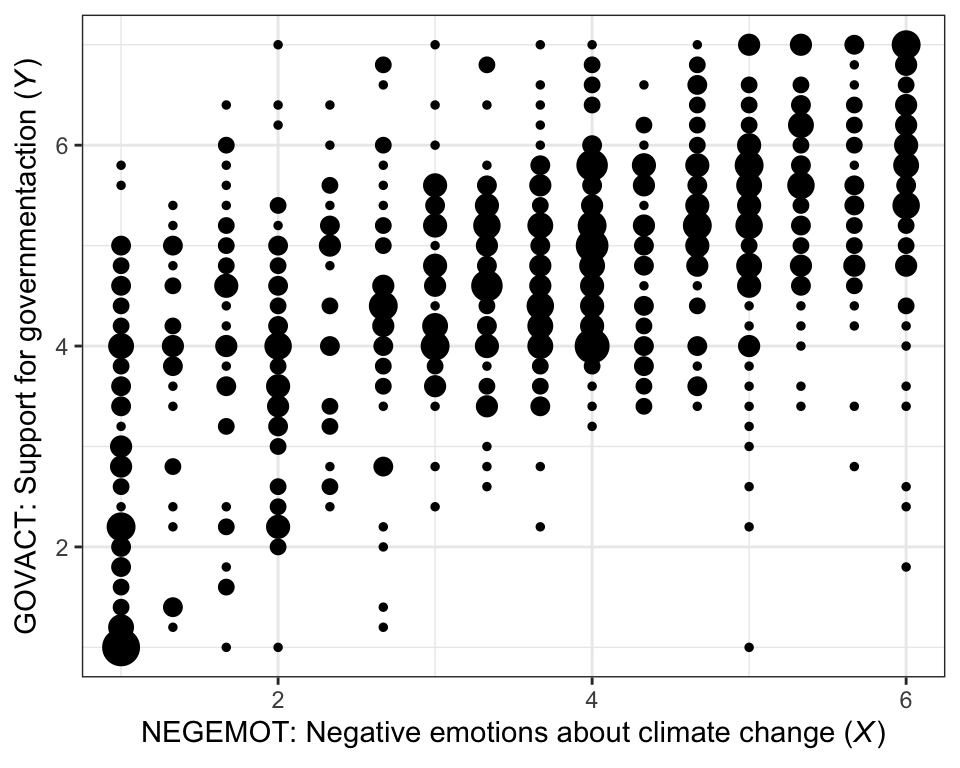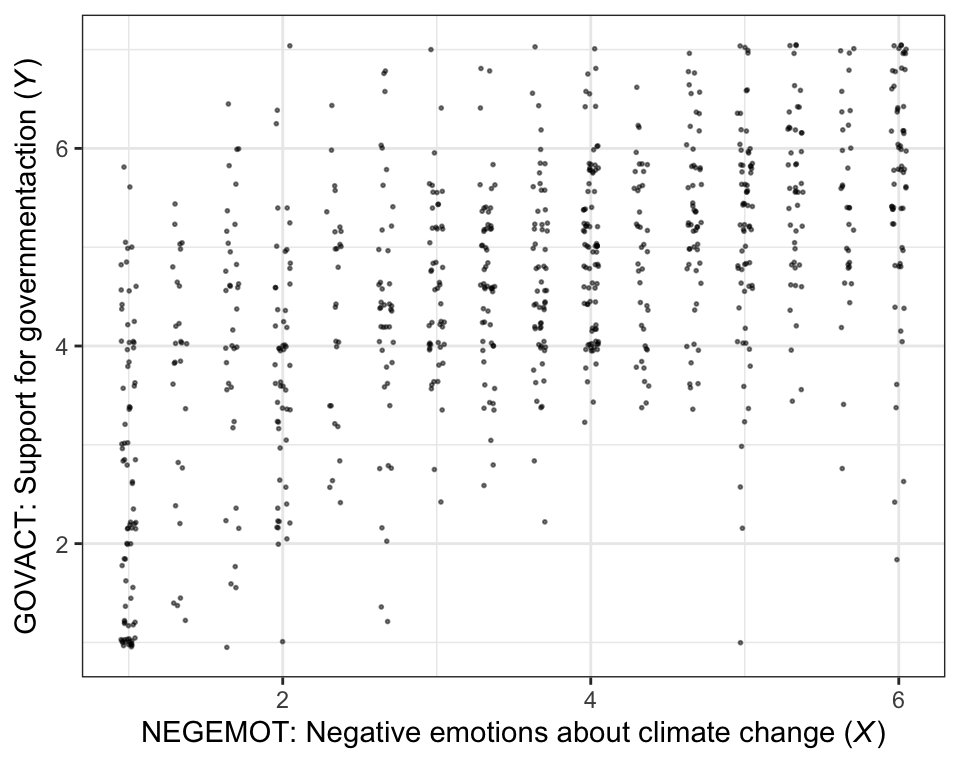## 2.1 Correlation and prediction

Here we load a couple necessary packages, load the data, and take a peek.

library(tidyverse)

glimpse(glbwarm)
#> Observations: 815
#> Variables: 7
#> $govact <dbl> 3.6, 5.0, 6.6, 1.0, 4.0, 7.0, 6.8, 5.6, 6.0, 2.6, 1.4, 5.6, 7.0, 3.8, 3.4, 4.2, 1.0, 2.6... #>$ posemot  <dbl> 3.67, 2.00, 2.33, 5.00, 2.33, 1.00, 2.33, 4.00, 5.00, 5.00, 1.00, 4.00, 1.00, 5.67, 3.00...
#> $negemot <dbl> 4.67, 2.33, 3.67, 5.00, 1.67, 6.00, 4.00, 5.33, 6.00, 2.00, 1.00, 4.00, 5.00, 4.67, 2.00... #>$ ideology <int> 6, 2, 1, 1, 4, 3, 4, 5, 4, 7, 6, 4, 2, 4, 5, 2, 6, 4, 2, 4, 4, 2, 6, 4, 4, 3, 4, 5, 4, 5...
#> $age <int> 61, 55, 85, 59, 22, 34, 47, 65, 50, 60, 71, 60, 71, 59, 32, 36, 69, 70, 41, 48, 38, 63, ... #>$ sex      <int> 0, 0, 1, 0, 1, 0, 1, 1, 1, 1, 1, 0, 1, 0, 1, 1, 1, 0, 0, 0, 0, 1, 1, 1, 1, 1, 1, 0, 0, 1...
#> $partyid <int> 2, 1, 1, 1, 1, 2, 1, 1, 2, 3, 2, 1, 1, 1, 1, 1, 2, 3, 1, 3, 2, 1, 3, 2, 1, 1, 1, 3, 1, 1... If you are new to tidyverse-style syntax, possibly the oddest component is the pipe (i.e., %>%). I’m not going to explain the %>% in this project, but you might learn more about in this brief clip, starting around minute 21:25 in this talk by Wickham, or in section 5.6.1 from Grolemund and Wickham’s R for Data Science. Really, all of chapter 5 of R4DS is great for new R and new tidyverse users, and their chapter 3 is a nice introduction to plotting with ggplot2. Here is our version of Figure 2.1. glbwarm %>% group_by(negemot, govact) %>% count() %>% ggplot(aes(x = negemot, y = govact)) + geom_point(aes(size = n)) + labs(x = expression(paste("NEGEMOT: Negative emotions about climate change (", italic("X"), ")")), y = expression(paste("GOVACT: Support for governmentaction (", italic("Y"), ")"))) + theme_bw() + theme(legend.position = "none")There are other ways to handle the overplotting issue, such as jittering. glbwarm %>% ggplot(aes(x = negemot, y = govact)) + geom_jitter(height = .05, width = .05, alpha = 1/2, size = 1/3) + labs(x = expression(paste("NEGEMOT: Negative emotions about climate change (", italic("X"), ")")), y = expression(paste("GOVACT: Support for governmentaction (", italic("Y"), ")"))) + theme_bw()The cor() function is a simple way to compute a Pearson’s correlation coefficient. cor(glbwarm$negemot, glbwarm$govact) #>  0.578 If you want more plentiful output, the cor.test() function returns a $$t$$-value, the degrees of freedom, the corresponding $$p$$-value and the 95% confidence intervals, in addition to the Pearson’s correlation coefficient. cor.test(glbwarm$negemot, glbwarm$govact) #> #> Pearson's product-moment correlation #> #> data: glbwarm$negemot and glbwarm\$govact
#> t = 20, df = 800, p-value <2e-16
#> alternative hypothesis: true correlation is not equal to 0
#> 95 percent confidence interval:
#>  0.530 0.622
#> sample estimates:
#>   cor
#> 0.578

To get the Bayesian version, we’ll open our focal statistical package, Bürkner’s brms. [And I’ll briefly note that you could also do many of these analyses with other packages, such as blavaan. I just prefer brms.]

library(brms)

We’ll start simple and just use the default priors and settings, but with the addition of parallel sampling via cores = 4.

model1 <-
brm(data = glbwarm, family = gaussian,
cbind(negemot, govact) ~ 1,
chains = 4, cores = 4)
print(model1)
#>  Family: MV(gaussian, gaussian)
#>   Links: mu = identity; sigma = identity
#>          mu = identity; sigma = identity
#> Formula: negemot ~ 1
#>          govact ~ 1
#>    Data: glbwarm (Number of observations: 815)
#> Samples: 4 chains, each with iter = 2000; warmup = 1000; thin = 1;
#>          total post-warmup samples = 4000
#>
#> Population-Level Effects:
#>                   Estimate Est.Error l-95% CI u-95% CI Eff.Sample Rhat
#> negemot_Intercept     3.56      0.05     3.45     3.67       3633 1.00
#> govact_Intercept      4.59      0.05     4.50     4.68       3647 1.00
#>
#> Family Specific Parameters:
#>                        Estimate Est.Error l-95% CI u-95% CI Eff.Sample Rhat
#> sigma_negemot              1.53      0.04     1.46     1.61       3603 1.00
#> sigma_govact               1.36      0.03     1.30     1.43       3558 1.00
#> rescor(negemot,govact)     0.58      0.02     0.53     0.62       3800 1.00
#>
#> Samples were drawn using sampling(NUTS). For each parameter, Eff.Sample
#> is a crude measure of effective sample size, and Rhat is the potential
#> scale reduction factor on split chains (at convergence, Rhat = 1).

Within the brms framework, $$\sigma$$ of the Gaussian likelihood is considered a family-specific parameter (e.g., there is no $$\sigma$$ for the Poisson distribution). When you have an intercept-only regression model with multiple variables, the covariance among their $$\sigma$$ parameters are correlations. Since our multivariate model is of two variables, we have one covariance for the $$\sigmas$$s, rescor(negemot,govact), which is our Bayesian analogue to the Pearson’s correlation.

To learn more about the multivariate syntax in brms, click here or code vignette("brms_multivariate").

But to clarify the output:

• ‘Estimate’ = the posterior mean, analogous to the frequentist point estimate
• ‘Est.Error’ = the posterior $$SD$$, analogous to the frequentist standard error
• ‘l-95% CI’ = the lower-level of the percentile-based 95% Bayesian credible interval
• ‘u-95% CI’ = the upper-level of the same